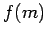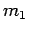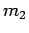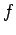Next: How to play poker Up: Probabilistic Encryption Previous: The inability to distinguish   Contents

## Semantic Security

Theorem 5.2 of the paper shows that there is no property of the plaintext message which can be efficiently estimated by looking at the ciphertext. Typical properties might be ``the last bit of the plaintext is 0'' or ``the number of 1's is twice as much as the number of 0's.'' In general, a property is defined in the paper as the value of a functionwhich takes a message as input and gives a number as output. Ifis constant for all, prediction ofis trivial. Similarly, ifis almost constant for almost all, there is a simple algorithm which will be close to right with high prob

We wish to show that, except in the special cases we've mentioned, there is no efficient algorithm which will predictfrom the ciphertext for. If there were, we could run our algorithm to estimateon the ciphertext from randomly generateduntil we found,on which the algorithm behaved differently. But this would contradict the result of the previous section.

[The paper points out that it is not assumed thatis an easily computable function. I think this is a minor issue. The theorem really discusses the capabilities of a an easily computable program for estimating.]Next: How to play poker Up: Probabilistic Encryption Previous: The inability to distinguish   Contents
Translated from LaTeX by Scott Sutherland
2002-12-14